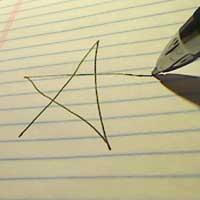# Stars and Asters

9 Nov

Some upcoming world flag tutorials are going to use two different types of stars, so I thought I’d spend some time explaining how they are made. The above sketch contains some sample code you can borrow for drawing stars.Here’s how I usually draw a star, when doodling. Maybe you do it the same way. This is the same kind of 5-pointed star that appears in the US flag. I start from the lower left, and then draw 5 lines in quick succession, connecting nearly opposite vertices. Typically, when doing a 5 pointed star, I connect every 2 points with a line. This only works if the number of points, and the number of points you are skipping (2 in this case) are relatively prime numbers. You can’t make a six-pointed star this way.

I’ve written a routine DrawStar, which generalizes this kind of drawing, so you can make a lot of different stars and spyrograph effects with it. In the first example, the two yellow stars on the left were drawn with it. Here’s the code:

```void drawStar(int nbrPoints, float cx, float cy, float odiam, int skipPoints )
{
float orad = odiam / 2.0;

pushMatrix();
translate(cx, cy);

float  a = TWO_PI / nbrPoints;

beginShape();
int  n = 0;
for (int i = 0; i < nbrPoints; ++i) {
vertex( cos( a * n) * orad, sin( a * n) * orad);
n += skipPoints;
}
endShape(CLOSE);
popMatrix();
}
```

To use this routine, you have to supply the following parameters:

• nbrPoints The number of points in the star (5 is a good choice, 6 is not so good)
• cx,cy The center coordinates of the star
• odiam The outer diameter (or width) of the star
• skipPoints The number of points to skip when drawing the star. It should be
relatively prime with nbrPoints if you want the classic star effect.The second way I have of drawing stars is slightly more complicated. I draw two lines for each point, in a sunburst-like pattern. To distinguish this second type of star from the first, I'm going to call this an "aster" which is latin for star (I haven't found a standard nomenclature for distinguishing the two methods).

The American (USA) flag contains stars of the first type, but the Australian flag contains asters. With asters, you can control both the outer and inner diameter, and achieve a lot of different kinds of stars, from spiny stars to fat bulging stars. In the first illustration, the 4 remaining yellow stars on the right are 5-pointed asters. The 7 and 5-pointed asters on the Australian flag happen to have an inner diameter which is 4/9 the outer diameter. It is this ratio that you pass as the final parameter to my drawAster routine, which is shown below.

```void drawAster(int nbrPoints, float cx, float cy, float odiam, float idiamRatio)
{
float orad = odiam / 2;

pushMatrix();
translate(cx, cy);

float  a = TWO_PI / nbrPoints;

beginShape();
for (int i = 0; i < nbrPoints; ++i) {
vertex( cos( a * i) * orad, sin( a * i) * orad);
vertex( cos( a * (i+.5)) * irad, sin( a * (i+.5)) * irad);
}
endShape(CLOSE);
popMatrix();
}
```

To use this routine, you have to supply the following parameters, which, with the exception of the last one, are the same as for my other star drawing routine:

• nbrPoints The number of points in the star (5 is a good choice, 6 is not so good)
• cx,cy The center coordinates of the star
• odiam The outer diameter (or width) of the star
• iratioRatio The ratio of the inner diameter to the outer diameter (e.g. .444 for the Australian flag stars).

The background stars in the first illustration are a variety of stars drawn using both routines, with copious use of random numbers. It is not hard to produce snow-flake like effects by changing the colors and the symmetry.

Tags: , ,